Next: Operators in quantum mechanics Up: Quantum Mechanics Previous: Quantum Mechanics

### Concept of wave function and related postulates

The wave function is the most important entity in quantum mechanics. The first postulate of quantum mechanics states that the state of a system is completely described by the wave function (also called the state function)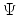(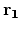,,....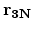, t) which is a function of the 3N spatial coordinates of the 3N particles constituting the system and time t. In general,may also depend on some intrinsic coordinates of the particles called spin coordinates. By saying completely described, what is meant is that the wave function contains all the information about the different properties of the system that may be experimentally determined.

How to elicit information out of the wave function is the subject matter of another postulate better known as the Born interpretation (courtesy Max Born) which is as follows:

The probability of finding a particle between x and x + dx, y and y + dy and between z and z + dz, or in other words inside a box of volume dV = dxdydz, is proportional to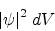where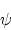is the wave function for the particle at that instant of time.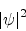is then called the probability density (i.e. probability per unit volume in 3 dimensions).

Since the particle is bound to be found somewhere, the probability of finding the particle over all space is given by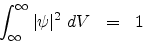Thenis said to be a normalized wave function.

If any wave function is not normalized then it can be made normalized by multiplying with a constant such that the above equation holds with the resulting new wave function.Next: Operators in quantum mechanics Up: Quantum Mechanics Previous: Quantum Mechanics
Abhijit Poddar
2007-09-27